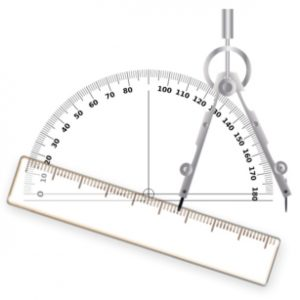The area of triangle PQR is 3√3 square centimetres. Angle PRQ is 60 degrees and RQ is 4 centimetres longer than PR.
Find the length of PQ.
Draw ΔPQR accurately.
Calculate all the angles in the triangle.

Tagged with: